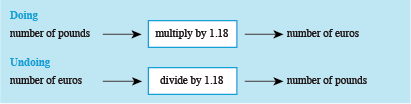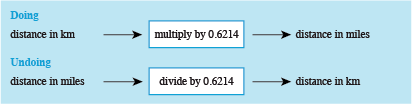Science, Maths & Technology

### Become an OU studentSucceed with maths: part 2

Start this free course now. Just create an account and sign in. Enrol and complete the course for a free statement of participation or digital badge if available.

# 4 Rearranging formulas

Rearranging a formula makes it possible to show the formula in a different way, whilst making sure the relationships between the various elements that it describes are not changed. If these relationships are changed, the formula will no longer work as expected. Thinking about rearranging using the ‘doing’ and ‘undoing’ technique from the last section will help to ensure that the relationships are maintained.

Instead of a using a set of instructions, the next example is from Week 3. Hopefully, you recognise this word formula:

How could a visitor from Europe use this word formula to convert euros into pounds, say, while shopping on holiday? They would need a formula for the number of pounds based on the number of euros. This can tackled by drawing the ‘doing and undoing’ diagrams for this situation.

In this case, the starting point for the doing diagram will be number of pounds on the left, the operation in the box will be multiply by 1.18 and output on the right will be number of euros (our answer). Therefore, to undo the operation in the box divide by 1.18. So, the diagrams will look like those shown in Figure 13.Figure 13 Doing and undoing diagrams for converting pounds to euros and euros to pounds

From the undoing diagram the new word formula can be built. This gives:

This just needs to be swapped around, so that the formula is shown in the conventional way with what you are calculating stated first. This results in:

Now use what you have learned here in this next activity.

## Activity 8 Miles and kilometres

Timing: Allow approximately 5 minutes

To change kilometres into miles, you can use the formula:

Starting with ‘distance in kilometres’, draw a doing diagram to show how to calculate the distance in miles. Then draw the undoing diagram and write down the formula for changing miles into kilometres.

Your formula should start ‘distance in kilometres = ...’.